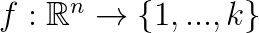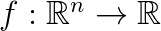Show Question

Classification
The computer is asked to specify which of k categories some input belongs to. The learning algorithm is usually asked to produce a function$f: \mathbb{R}^n \rightarrow \{1,...,k\}$. When y = f(x), the model assigns an input described by vector x to a category identified by numeric code y. Another type of algorithm might instead output a probability distribution over classes, instead of just a single result.
Classification with missing inputs
When some of the inputs can be missing, the learning algorithm, instead of defining a single function taking the input vector, it must define a set of functions, one function for each variantion of missing inputs. This type of situation arrises frequently in medical diagnostics, as many medical tests are expensive or invasive. One way to efficiently define all these functions is to learn a probability distribution over all variables, then obtain specific functions my marginalizing out the missing variables. Thus, with n inputs, 2^n functions can be obtained. Many other tasks described below can also be generalized to work with missing inputs.
Regression
The computer is asked to predict a numerical value given some input. To solve this task, the learning algorithm must output a function$f: \mathbb{R}^n \rightarrow \mathbb{R}$
Transcription
The machine learning system is asked to observe a relatively unstructured representation of some kind of information and to convert it to discrete textual/binary form. For example, convert an image of text to a sequence of characters; convert a recording of speech to text.
Machine Translation
The input is already a sequence of symbols in some language, and the learning algorithm is asked to convert this into a sequence of symbols in another language. This is commonly applied to natural languages. 5
Structured Output
Structured output tasks are any task where the output is a vector (or any other multi-valued data structure) where there is important relationships between the elements. For example, parsing natural language text into a structure describing its grammar. The category of structured output is broad and subsumes transcription and translation tasks. Other examples include: annotating roads in maps, captioning images. The output of these algorithms is tightly interrelated values (for the caption case, the output must be a valid sentence).
Anomaly detection
The computer sifts through a set of events or objects and flags some of them as being unusual or atypical.
Synthesis and sampling
The machine learning algorithm is asked to generate new examples that are similar to those in the training data. It is useful when generating large volumes of content by hand would be expensive, boring, or require too much time. Examples: video games generating textures for objects and landscapes.
Imputation of missing values
The machine learning algorithm is given an example vector, but with some entries missing. The task is to predict the values of the missing entries.
Denoising
The machine learning algorithm is given a corrupted example (a vector), obtained by an unknown corruption process from a clean example. The algorithm must predict the clean example from the corrupted version. More generally, it must predict the conditional probability distribution p(non-corrupted case | corrupted case).
Density estimation (probability mass function estimation)
The machine learning algorithm must learn the probability density function of the space from which the multi-dimensional examples are drawn from. Knowing the probability distribution can help with solving some of the other problems described above.